# rsparse v0.3.3.2

0

0th

Percentile

## Statistical Learning on Sparse Matrices

Implements many algorithms for statistical learning on sparse matrices - matrix factorizations, matrix completion, elastic net regressions, factorization machines. Also 'rsparse' enhances 'Matrix' package by providing methods for multithreaded <sparse, dense> matrix products and native slicing of the sparse matrices in Compressed Sparse Row (CSR) format. List of the algorithms for regression problems: 1) Elastic Net regression via Follow The Proximally-Regularized Leader (FTRL) Stochastic Gradient Descent (SGD), as per McMahan et al(, <doi:10.1145/2487575.2488200>) 2) Factorization Machines via SGD, as per Rendle (2010, <doi:10.1109/ICDM.2010.127>) List of algorithms for matrix factorization and matrix completion: 1) Weighted Regularized Matrix Factorization (WRMF) via Alternating Least Squares (ALS) - paper by Hu, Koren, Volinsky (2008, <doi:10.1109/ICDM.2008.22>) 2) Maximum-Margin Matrix Factorization via ALS, paper by Rennie, Srebro (2005, <doi:10.1145/1102351.1102441>) 3) Fast Truncated Singular Value Decomposition (SVD), Soft-Thresholded SVD, Soft-Impute matrix completion via ALS - paper by Hastie, Mazumder et al. (2014, <arXiv:1410.2596>) 4) Linear-Flow matrix factorization, from 'Practical linear models for large-scale one-class collaborative filtering' by Sedhain, Bui, Kawale et al (2016, ISBN:978-1-57735-770-4) 5) GlobalVectors (GloVe) matrix factorization via SGD, paper by Pennington, Socher, Manning (2014, <https://www.aclweb.org/anthology/D14-1162>) Package is reasonably fast and memory efficient - it allows to work with large datasets - millions of rows and millions of columns. This is particularly useful for practitioners working on recommender systems.

rsparse is an R package for statistical learning primarily on sparse matrices - matrix factorizations, factorization machines, out-of-core regression. Many of the implemented algorithms are particularly useful for recommender systems and NLP.

On top of that we provide some optimized routines to work on sparse matrices - multithreaded matrix multiplications and improved support for sparse matrices in CSR format (Matrix::RsparseMatrix).

We've paid some attention to the implementation details - we try to avoid data copies, utilize multiple threads via OpenMP and use SIMD where appropriate. Package allows to work on datasets with millions of rows and millions of columns.

### Support

Please reach us if you need commercial support - hello@rexy.ai.

# Features

### Classification/Regression

1. Follow the proximally-regularized leader which llows to solve very large linear/logistic regression problems with elastic-net penalty. Solver use with stochastic gradient descend with adaptive learning rates (so can be used for online learning - not necessary to load all data to RAM). See Ad Click Prediction: a View from the Trenches for more examples.
• Only logistic regerssion implemented at the moment
• Native format for matrices is CSR - Matrix::RsparseMatrix. However common R Matrix::CpasrseMatrix (dgCMatrix) will be converted automatically.
2. Factorization Machines supervised learning algorithm which learns second order polynomial interactions in a factorized way. We provide highly optimized SIMD accelerated implementation.

### Matrix Factorizations

1. Vanilla Maximum Margin Matrix Factorization - classic approch for "rating" prediction. See WRMF class and constructor option feedback = "explicit". Original paper which indroduced MMMF could be found here.
•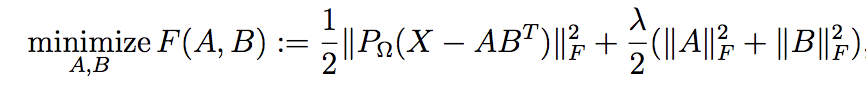2. Weighted Regularized Matrix Factorization (WRMF) from Collaborative Filtering for Implicit Feedback Datasets. See WRMF class and constructor option feedback = "implicit". We provide 2 solvers:
1. Exact based of Cholesky Factorization
2. Approximated based on fixed number of steps of Conjugate Gradient. See details in Applications of the Conjugate Gradient Method for Implicit Feedback Collaborative Filtering and Faster Implicit Matrix Factorization.
3.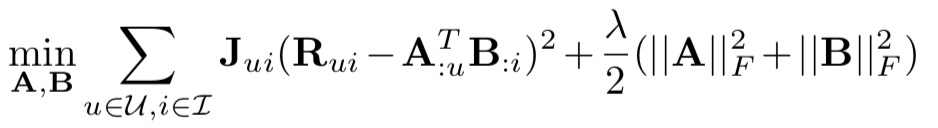3. Linear-Flow from Practical Linear Models for Large-Scale One-Class Collaborative Filtering. Algorithm looks for factorized low-rank item-item similarity matrix (in some sense it is similar to SLIM)
•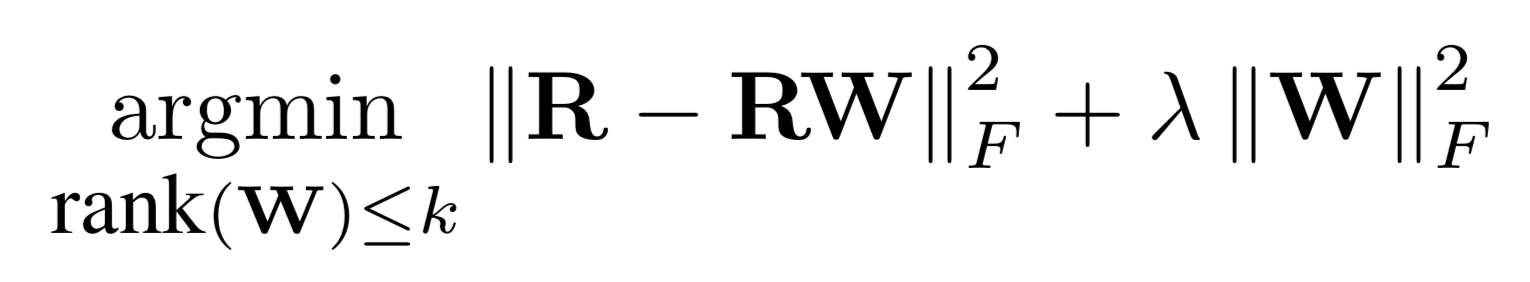4. Fast Truncated SVD and Truncated Soft-SVD via Alternating Least Squares as described in Matrix Completion and Low-Rank SVD via Fast Alternating Least Squares. Works for both sparse and dense matrices. Works on float matrices as well! For certain problems may be even faster than irlba package.
•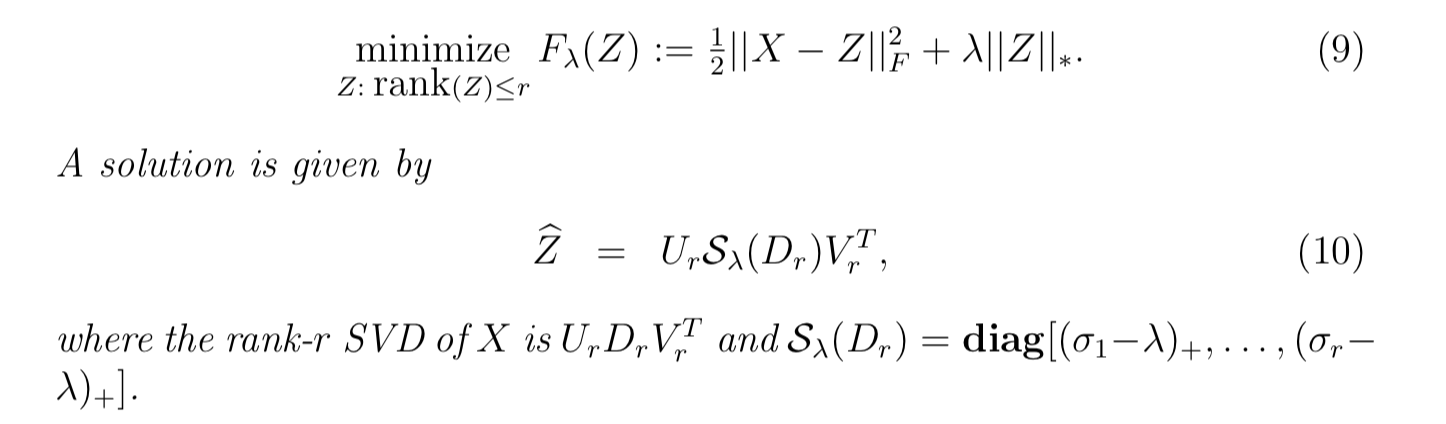5. Soft-Impute via fast Alternating Least Squares as described in Matrix Completion and Low-Rank SVD via Fast Alternating Least Squares.
•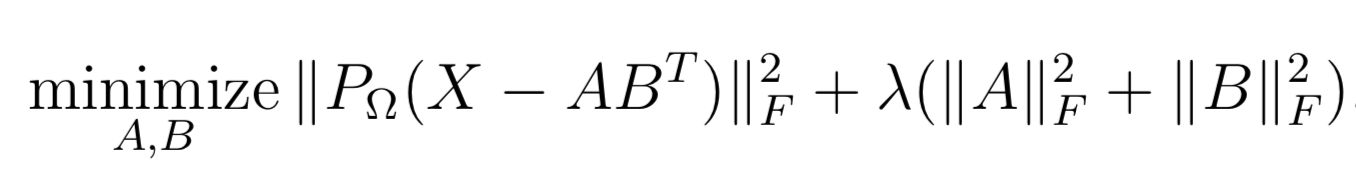• with a solution in SVD form6. GloVe as described in GloVe: Global Vectors for Word Representation.
• This is usually used to train word embeddings, but actually also very useful for recommender systems.

### Optimized matrix operations

1. multithreaded %*% and tcrossprod() for <dgRMatrix, matrix>
2. multithreaded %*% and crossprod() for <matrix, dgCMatrix>
3. natively slice CSR matrices (Matrix::RsparseMatrix) without converting them to triplet / CSC

# Installation

Most of the algorithms benefit from OpenMP and many of them could utilize high-performance implementation of BLAS. If you want make maximum out of the package please read the section below carefuly.

It is recommended to:

1. Use high-performance BLAS (such as OpenBLAS, MKL, Apple Accelerate).
2. Add proper compiler optimizations in your ~/.R/Makevars. For example on recent processors (with AVX support) and complier with OpenMP support following lines could be a good option:
 CXX11FLAGS += -O3 -march=native -mavx -fopenmp -ffast-math
CXXFLAGS   += -O3 -march=native -mavx -fopenmp -ffast-math


If you are on Mac follow instructions here. After installation of clang4 additionally put PKG_CXXFLAGS += -DARMA_USE_OPENMP line to your ~/.R/Makevars. After that install rsparse in a usual way.

# Materials

Note that syntax is these posts/slides is not up to date since package was under active development

1. Slides from DataFest Tbilisi(2017-11-16)
2. Introduction to matrix factorization with Weighted-ALS algorithm - collaborative filtering for implicit feedback datasets.
3. Music recommendations using LastFM-360K dataset
• evaluation metrics for ranking
• setting up proper cross-validation
• possible issues with nested parallelism and thread contention
• making recommendations for new users
• complimentary item-to-item recommendations
4. Benchmark against other good implementations

Here is example of rsparse::WRMF on lastfm360k dataset in comparison with other good implementations:# Configure

Generate configure:

autoconf configure.ac > configure && chmod +x configure


## Functions in rsparse

 Name Description WRMF Weighted Regularized Matrix Facrtorization for collaborative filtering soft_impute SoftImpute/SoftSVD matrix factorization slice CSR Matrices Slicing train_test_split Creates cross-validation set from user-item interactions GloVe Creates Global Vectors matrix factorization model. LinearFlow Creates Linear-FLow model for one-class collaborative filtering FTRL Creates FTRL proximal logistic regression model. FactorizationMachine Creates FactorizationMachine model. PureSVD Soft-SVD decompomposition metrics Ranking Metrics for Top-K Items movielens100k MovieLens 100K Dataset detect_number_omp_threads Detects number of OpenMP threads in the system matmult Multithreaded Sparse-Dense Matrix Multiplication No Results!